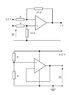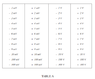# Calculating the unknown voltages in ideal op-amp circuits

• Engineering
• peasngravy
peasngravy
Homework Statement
FIGURE 1 shows two amplifier circuits, each of which has one unknown
voltage (shown highlighted). Select, from TABLE A, the most
appropriate value for the unknown voltages for each circuit. Assume that
the op-amps are ideal and that the magnitude of their output voltages is
less than their maximum peak output voltage swing (VOM).
Relevant Equations
V+ = V1/(R+10R)

(V2 - V-)/R = -(Vo - V-)/10RHi - I have made an attempt at both of these circuits, but I am not 100% sure I am correct. Would it be possible to check if I have this right, and if not, please give me some pointers as to where I have gone wrong? Thanks

First op-amp
Given data:
Vo = 5V
I have called the voltage feeding into the non-inverting input V1 = 0.5V
voltage feeding into the inverting input = V2

For non inverting input V+ = V1/(1+10)

For inverting input V- :
(V2 - V-)/R= -(Vo - V-)/10R
V2 - V- =(-Vo + V-)/10
10(V2 - V-)-V- = -Vo
10V2 - 11V- = -Vo
Plug in values
(10*0.5) - 11V- = -5
-11V- = -10
V- =10/11 = 0.909V

As it is an ideal op-amp:

V+ = V- = 0.909V

Therefore:

V+ =V1/(10+1)

V1 = V+ * 11
V1 = 0.909*11 = 10V

Second op-amp

At non-inverting input:

V+ =Vo/(R+R)
V+=12/2=6V

As it is an ideal op-amp:

V+ = V- =6V
Due to short from inverting input to output, output voltage (V) is 6V

Homework Helper
Gold Member
For non inverting input V+ = V1/(1+10)
You are relating the non-inverting "feeding" voltage (V1) to the actual voltage at the non-inverting node (V+) right?
In that case, you have incorrectly applied the voltage divider formula here.

peasngravy
You are relating the non-inverting "feeding" voltage (V1) to the actual voltage at the non-inverting node (V+) right?
In that case, you have incorrectly applied the voltage divider formula here.

Yep that's what I was trying to do. What have I done wrong with the formula?

Homework Helper
Gold Member
Yep that's what I was trying to do. What have I done wrong with the formula?
Your V+ is the voltage across 10R.
The total series resistance is 11R.
How are V1 and V+ related then?

peasngravy
Your V+ is the voltage across 10R.
The total series resistance is 11R.
How are V1 and V+ related then?

So should it be
(0.5-V2)/R = (V2-5)/10R
?

Homework Helper
Gold Member
No.
You have input voltage V1 and two series resistances R and 10R forming a voltage divider.
What fraction of V1 is dropped across 10R?

You have calculated that fraction. You need to find V1 from that fraction.

peasngravy
No.
You have input voltage V1 and two series resistances R and 10R forming a voltage divider.
What fraction of V1 is dropped across 10R?

You have calculated that fraction. You need to find V1 from that fraction.

I see - so it is 10/11?

Homework Helper
Gold Member
I see - so it is 10/11?
Yes.

peasngravy
Thank you - so

V1= 10/(11*0.91) = 0.99V?

Homework Helper
Gold Member
V1= 10/(11*0.91) = 0.99V?
Sure, but if you keep all the terms in fraction form till the end, you will get V1= 1V, which is the accurate answer.

peasngravy
Thank you so much for helping me to understand.

Does the second op-amp look Ok to you?

Homework Helper
Gold Member
Does the second op-amp look Ok to you?
Yes 6V looks correct to me.

•peasngravy
peasngravy
•